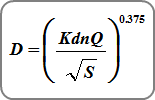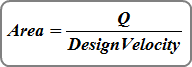# Automated Design

Contents

This software has certain automated design features. Basically, if a particular data item is left blank, for example the Pipe Rise, the program will design it for you.

As shown on the Pipe Design tab, Stormwater Studio offers various design options (Pipes only) regardless of which calculation option you use “Analysis & Design”, or “Full Design”. They are:

1. Reset Pipe Sizes
2. Reset Invert Elevations
3. Reset Pipe Sizes and Reset Invert Elevations

When designing, the program uses the following form of Manning’s equation.Where:
D = pipe diameter in ft (m)
n = Manning’s n-value
Q = line discharge in cfs (cms)
S = slope of the invert in ft/ft (m/m)
Kd = 2.16 (3.20)

This procedure assumes that the pipe is flowing full and that the slope of the invert is equal to the slope of the Energy Grade Line.

Remember, the S term, or slope, in Manning’s equation, in its traditional use, is the slope of the energy grade line. Not the slope of the invert. In real world conditions, the slope of the invert rarely coincides with the energy grade line.

## Reset Pipe Sizes

When this option has been checked On, or if any pipe Rise & Span has been set to zero, Stormwater Studio will automatically compute a pipe size by first computing the required cross-sectional area:Where:
Q = flow rate
Design Velocity = Minimum Velocity selected on the Pipe Design tab

It then selects an available pipe size whose area matches “Area”. It only chooses commercially available sizes and within the minimum and maximum sizes specified by you. Commercially available sizes are assumed to be 2 to 36 inches in 3-inch increments and 42 to 102 inches in 6-inch increments. See Programmed Pipe Sizes for a complete list including metric sizes.

When a specific pipe size is not available, the program will select the next smaller size. For example, if the theoretical size is 25.5 inches, the software will round down and select the 24-inch. This way the Minimum Velocity is maintained.

## Reset Invert Elevations

When this option has been checked On or when an invert elevation for a Line has been set to zero, Stormwater Studio simply uses the above equation, setting the velocity equal to the Minimum Velocity, and solves for S. It then sets the invert elevations accordingly. If the resulting slope, S, is less than the “Minimum Slope”, then S is reset to the Minimum Slope and a new pipe size will be selected at the new slope, using Manning’s equation.

If Follow Ground Surface has been checked On as a calculation option, then the slope is set initially to match the surface grade. If the slope exceeds the Maximum Slope, it is reset to the Maximum Slope.

If both of the above Reset options have been checked On, Stormwater Studio first selects an available pipe size whose area matches “Area”. With that chosen pipe size, it sets the inverts using the procedure described above.

Note that the final velocity will not always be exactly equal to the Minimum Velocity”. This is due to the affects of the downstream HGL after the design is complete.